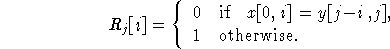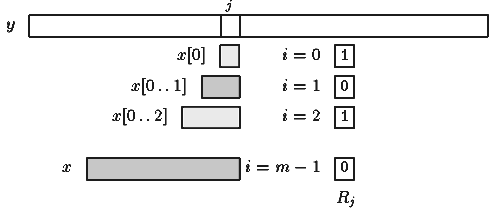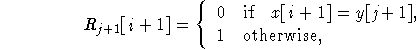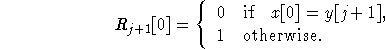Next: Morris-Pratt algorithm Up: ESMAJ Prev: Karp-Rabin algorithm

# Shift Or algorithm

Main features
• uses bitwise techniques;
• efficient if the pattern length is no longer than the memory-word size of the machine;
• preprocessing phase in O(m +) time and space complexity;
• searching phase in O(n) time complexity (independent from the alphabet size and the pattern length);
• adapts easily to approximate string matching.
Description

The Shift Or algorithm uses bitwise techniques. Let R be a bit array of size m. Vector Rj is the value of the array R after text character y[j] has been processed (see figure 5.1). It contains informations about all matches of prefixes of x that end at position j in the text for 0 < im-1:Figure 5.1: Meaning of vector Rj.

The vector Rj+1 can be computed after Rj as follows. For each Rj[i]=0:andIf Rj+1[m-1]=0 then a complete match can be reported.

The transition from Rj to Rj+1 can be computed very fast as follows: For each c in, let Sc be a bit array of size m such that: for 0i < m-1, Sc[i]=0 iff x[i]=c.

The array Sc denotes the positions of the character c in the pattern x. Each Sc can be preprocessed before the search. And the computation of Rj+1 reduces to two operations, shift and or: Rj+1=SHIFT(Rj) OR Sy[j+1]

Assuming that the pattern length is no longer than the memory-word size of the machine, the space and time complexity of the preprocessing phase is O(m+).

The time complexity of the searching phase is O(n), thus independent from the alphabet size and the pattern length.

The C code
```  int preSo(char *x, int m, unsigned int S[]) {
unsigned int j, lim;
int i;
for (i = 0; i < ASIZE; ++i)
S[i] = ~0;
for (lim = i = 0, j = 1; i < m; ++i, j <<= 1) {
S[x[i]] &= ~j;
lim |= j;
}
lim = ~(lim>>1);
return(lim);
}

void SO(char *x, int m, char *y, int n) {
unsigned int lim, state;
unsigned int S[ASIZE];
int j;
if (m > WORD)
error("SO: Use pattern size <= word size");

/* Preprocessing */
lim = preSo(x, m, S);

/* Searching */
for (state = ~0, j = 0; j < n; ++j) {
state = (state<<1) | S[y[j]];
if (state < lim)
OUTPUT(j - m + 1);
}
}

```
The example

Searching phaseAs R12=0 it means that an occurrence of x has been found at position 12-8+1=5.

Sorry the new example is not ready... See the Java applet.

References
• BAEZA-YATES, R.A., GONNET, G.H., 1992, A new approach to text searching, Communications of the ACM . 35(10):74-82.
• BAEZA-YATES R., NAVARRO G., RIBEIRO-NETO B., 1999, Indexing and Searching, in Modern Information Retrieval, Chapter 8, pp 191-228, Addison-Wesley.
• CROCHEMORE, M., LECROQ, T., 1996, Pattern matching and text compression algorithms, in CRC Computer Science and Engineering Handbook, A. Tucker ed., Chapter 8, pp 162-202, CRC Press Inc., Boca Raton, FL.
• WU, S., MANBER, U., 1992, Fast text searching allowing errors, Commun. ACM. 35(10):83-91.

Please note that this page has been translated into Ukrainian by Vasil Vashenko and into Danish by Nastasya Zemina.Next: Morris-Pratt algorithm Up: ESMAJ Prev: Karp-Rabin algorithm

e-mails: {Christian.Charras, Thierry.Lecroq}@laposte.net
Tue Jan 14 15:03:31 MET 1997